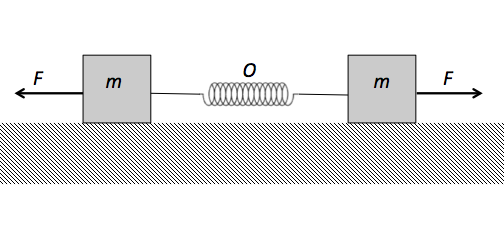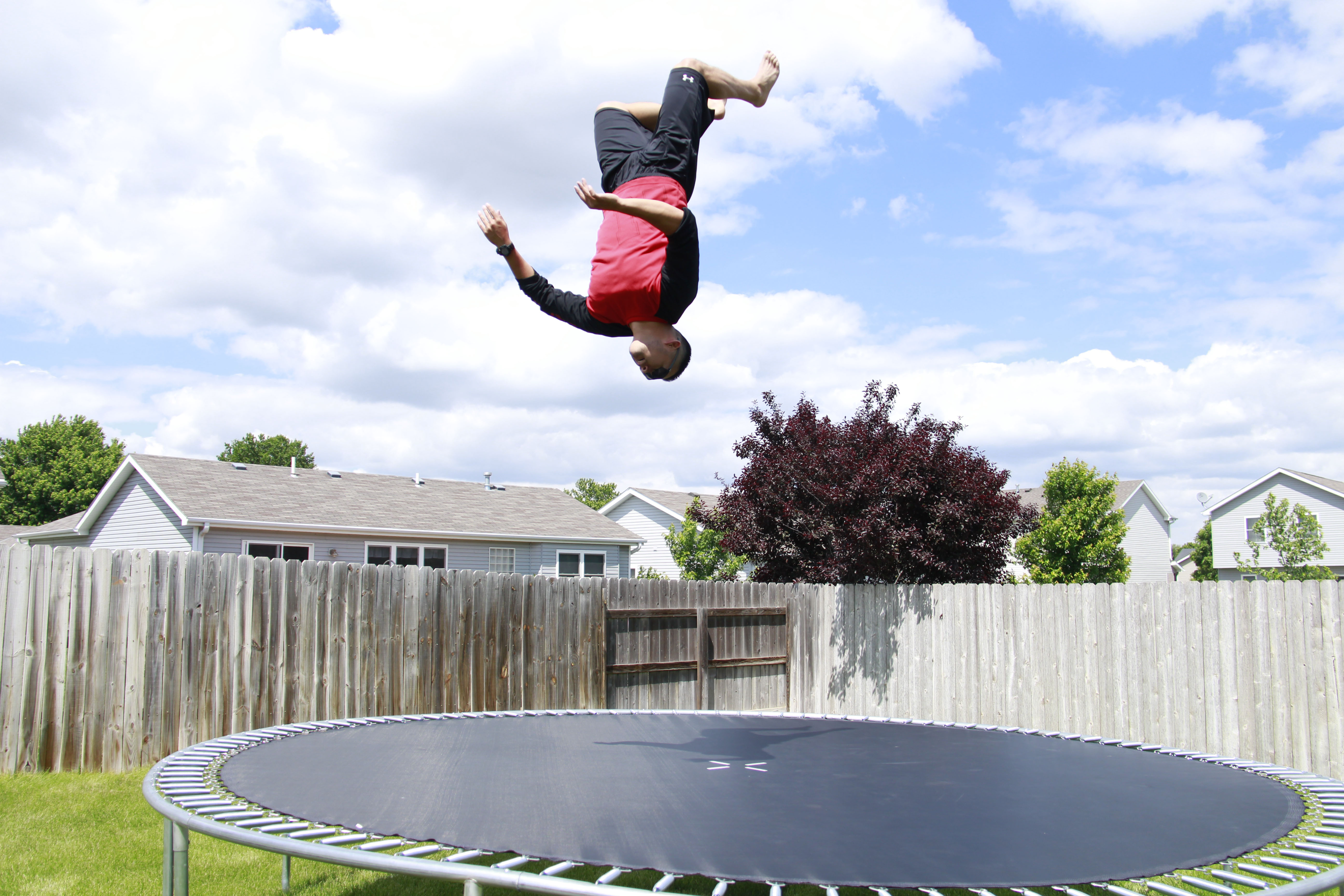Classical Mechanics

# Simple Harmonic Motion - Problem Solving

A body executing linear simple harmonic motion has a velocity of $7.0 \text{ cm/s}$ when its displacement is $2.0 \text{ cm},$ and a velocity of $2.0 \text{ cm/s}$ when its displacement is $7.0 \text{ cm}.$ What is the square of the amplitude of oscillation?

A body executing linear simple harmonic motion has a velocity of $8.0 \text{ cm/s}$ when its displacement is $3.0 \text{ cm},$ and a velocity of $3.0 \text{ cm/s}$ when its displacement is $8.0 \text{ cm}.$ If the mass of the oscillator is $5.0 \text{ kg},$ what is the approximate total energy of the oscillator?

Not every oscillation in nature is a harmonic oscillation - in this problem, we will examine a non-harmonic oscillation. Suppose we had a rubber ball with a perfect coefficient of restitution so that, when dropped, it would always return to the same height. The period of the bouncing is the time $T$ between successive bounces and the amplitude $A$ of this non-linear oscillation is the maximum height of the ball above the floor. For a harmonic oscillator, the period and amplitude are independent, but not so here. Instead, there's a relation between them: $T$ proportional to $A^w$. Find $w$.Two blocks, each of mass $m = 2.0 \text{ kg},$ are connected by a spring of force constant $k = 3.0 \text{ N/m}$ and placed on a horizontal frictionless surface, as shown in the above diagram. If an equal force of $F = 2.0 \text{ N}$ is applied to each block in the direction of arrow, what is the approximate time period of the system when the force is removed?You bounce on a huge trampoline and start oscillating up and down with a period of $3~\mbox{s}$. After a long while, you come to rest but the trampoline below you is displaced below its natural height. How much is the trampoline bent in m?

Details and assumptions

• Model the trampoline as a spring.
×